### LIMITS OF FUNCTIONS AS X APPROACHES INFINITY

The following problems require the algebraic computation of limits of functions as x approaches plus or minus infinity. Most problems are average. A few are somewhat challenging. All of the solutions are given WITHOUT the use of L'Hopital's Rule. If you are going to try these problems before looking at the solutions, you can avoid common mistakes by giving careful consideration to the formsandduring the computations of these limits. Initially, many students INCORRECTLY conclude thatis equal to 1 , or that the limit does not exist, or isor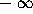. Many also conclude thatis equal to 0 . In fact, the formsandare examples of indeterminate forms. This simply means that you have not yet determined an answer. Usually, these indeterminate forms can be circumvented by using algebraic manipulation. Such tools as algebraic simplification and conjugates can easily be used to circumvent the formsandso that the limit can be calculated.
• PROBLEM 1 : Compute.

• PROBLEM 2 : Compute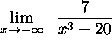.

• PROBLEM 3 : Compute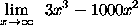.

• PROBLEM 4 : Compute.

• PROBLEM 5 : Compute.

• PROBLEM 6 : Compute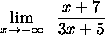.

• PROBLEM 7 : Compute.

• PROBLEM 8 : Compute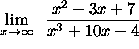.

• PROBLEM 9 : Compute.

• PROBLEM 10 : Compute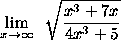.

• PROBLEM 11 : Compute.

• PROBLEM 12 : Compute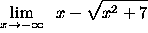.

• PROBLEM 13 : Compute.

• PROBLEM 14 : Compute.

• PROBLEM 15 : Compute.

• PROBLEM 16 : Compute limit (x to -infinity) cos [ x/(x^2+10) + pi/3 ] .

• PROBLEM 17 : Compute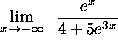.

• PROBLEM 18 : Compute.

• PROBLEM 19 : Compute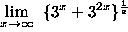.